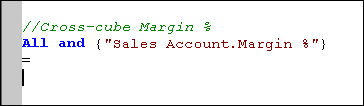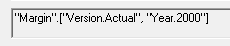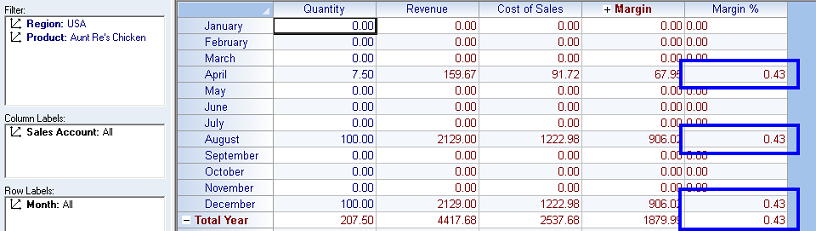Cross-Cube FormulaAs noted earlier, a cross-cube formula can be used to calculate values in a Cube using data from another Cube or even two or more Cubes. For example, Cubes A and B contain sales data for Year 1 and Year 2 (Year 2 being current year). Cube C might contain forecasts for Year 3. A cross-cube formula, created in Cube C, could use values in Cubes A and B to average their sales data and dynamically calculate a percentage-based increase in sales, showing results in Cube C. The fact that the calculations are dynamic (true for all PowerOLAP formula calculations) means that changing a variable will automatically recalculate the formula driving the forecast in Year 3 (Cube C), therefore, forecasts would be updated as new “Actuals” are recorded for Year 2 (Cube B).

Another example worth mentioning—particularly beneficial for multinational companies—would be the use of a Cube that stores monthly foreign exchange calculations. One could multiply the data in this Cube by the figures in a Financials cube, where company units report in local currency, to determine all figures, by month, in US dollars, back in the Financials cube.TipThe use of cross-cube formulas can dramatically improve performance, enabling you to calculate values only for certain Member intersection points and show them in a “standalone” Cube, rather than design a single Cube that performs many calculations across all Member intersection points.

The following example is a cross-cube formula that will, again, yield figures for Margin % figures in the Sales cube—but this time based on a calculation from Margin % in the Margin cube.

In this case, we must account for the fact that the Reference cube (Margin) has more Dimensions than the Sales cube, where the formula results will be written. (This is instructive because the difference in dimensionality is often the case in cross-cube formulas.)

 Cube Dimension Members Margin cube VersionYearRegionProductMonthMargin Account Sales cube RegionProductMonthSales Account

Our formula must instruct exactly which Members of the “extra Dimensions”—in Margin—to use in our calculation. Also, we need to indicate that Margin % in Sales will be based on Margin % in Margin.

1.   Open the Sales cube and click Formula to open the Formula Editor dialog.

In the Formula dialog box (for the Sales cube), the formula will include the same LHS statement for Margin % in Sales that we used in the last formula—i.e., the destination of the formula results is the same.

2.   Copy and paste the LHS of the formula earlier for Margin % then type in the equals sign after ( = ).To construct the RHS, referencing the Margin cube, we will now specify the Members from the Version and Year dimensions on which to base the calculation. (This will address the “dimensionality difference” we need to account for.) Since we want the Sales cube’s calculations to be based on Actuals for 2000, we can make the appropriate selections in the Build Cube Reference dialog box.

3.   Click on the Build Cube Reference button.

4.   Select the Margin cube from the Cubes drop-down—since this is a cross-cube formula, with data originating in that Cube.

5.   Click on Version in the Dimension list box on the left,
then select Actual in the Member list on the right.

6.   Next, click on Year in the Dimension list box,
then select 2000 in the Member list.

The grayed-out formula box at the bottom of the dialog box will, at this point, look as follows:[Note that the “originating Cube”—in this case, Margin, is indicated first—circled in the above figure.]

Now, logically, we need to specify that we want Margin % from the Margin Account dimension to be used for the calculation of Sales cube’s Margin %.

7.   Click on Margin Account in the Dimension list box,
then select Margin % in the Member list.

[Note that we will not need to specify the Dimensions that are common to the two Cubes—Region, Product and Month—thus signifying that all corresponding values apply between the two Cubes for these Dimensions.]

8.   Click OK and return to the Formulas dialog box.
Type a semi-colon (;) at the end of the formula.
The dialog box appears as follows:Now the Margin % formula, which brings Margin % from one Cube to another, is complete.This is a simple example of a cross-cube formula because it brings over the same figures in one Cube, Margin, to another Sales. Still, it is illustrative of how to proceed when the two Cubes have different dimensional structures. Furthermore, from this example you can easily grasp how you might perform other calculations on “originating” data (data in the first Cube).